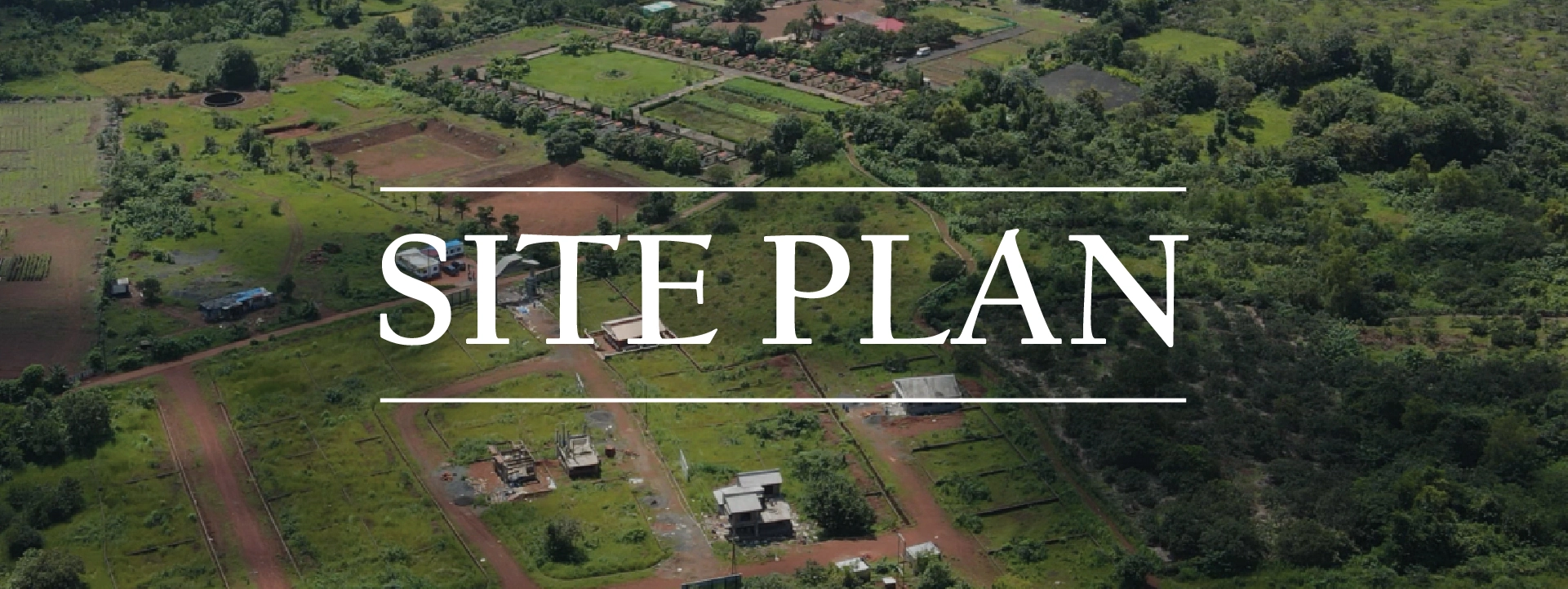Select Page### D (626 Sq. Ft.)Phase – I

Bungalow NameBungalow Area in (Sq. Ft.)Plot Area in (Sq. Ft.)
B-5513182529.54

### Total Plots =11

1 BHK Ground Floor Structure (626 Sq. Ft.)
Plot Area Starting From 1959 Sq. Ft. to 2917 Sq. Ft.
Total Units: 11

Plans are subject to revisions

### E-HR (E)(769 Sq. Ft.)Phase – I

Bungalow NameBungalow Area in (Sq. Ft.)Plot Area in (Sq. Ft.)
E-547792152.80
E-697792195.86
E-717792249.68
E-767792152.80
E-807792152.80
E-887792152.80
E-947792152.80

### Total Plots =07

2 BHK Ground Floor Structure HR (E/W) (769 Sq. Ft.)
Plot Area Starting From 2152 Sq. Ft. to 2680 Sq. Ft.
Total Units: 08

Plans are subject to revisions

### E-HR (W)(769 Sq. Ft.)Phase – I

Bungalow NameBungalow Area in (Sq. Ft.)Plot Area in (Sq. Ft.)
E-877792152.80

### Total Plots =01

2 BHK Ground Floor Structure HR (E/W) (769 Sq. Ft.)
Plot Area Starting From 2152 Sq. Ft. to 2680 Sq. Ft.
Total Units: 08

Plans are subject to revisions

### E-VR (E)(769 Sq. Ft.)Phase – I

Bungalow NameBungalow Area
in (Sq. Ft.)
Plot Area
in (Sq. Ft.)
A-579902421.90
A-96/A9902723.29
A-96/B9902228.15
A-100/A9902809.40
A-100/B9902034.40

### Total Plots =02

2 BHK Ground Floor Structure HR (E/W) (769 Sq. Ft.)
Plot Area Starting From 2152 Sq. Ft. to 2195 Sq. Ft.
Total Units: 04

Plans are subject to revisions

### E-VR (W)(769 Sq. Ft.)Phase – I

Bungalow NameBungalow Area in (Sq. Ft.)Plot Area in (Sq. Ft.)
A-6410272195.86
A-6610272131.27
A-8110272195.86
A-8310271959.05

### Total Plots =02

2 BHK Ground Floor Structure HR (E/W) (769 Sq. Ft.)
Plot Area Starting From 2152 Sq. Ft. to 2195 Sq. Ft.
Total Units: 04

Plans are subject to revisions

### A-HR (W)(972 Sq. Ft.)Phase – I

Bungalow NameBungalow Area
in (Sq. Ft.)
Plot Area
in (Sq. Mt.)
E-587792195.86
E-917792152.80

### Total Plots =02

2 BHK Ground + 1 Structure HR (W) (972 Sq. Ft.)
Plot Area Starting From 2195 Sq. Ft. to 3928 Sq. Ft.
Total Units: 02

Plans are subject to revisions

### Total Plots =05

2 BHK Ground + 1 Structure HR (E) (978 Sq. Ft.)
Plot Area Starting From 1765 Sq. Ft. to 2809 Sq. Ft.
Total Units: 05

Plans are subject to revisions

### Total Plots =06

2 BHK Ground + 1 Structure VR (E/W) (1017 Sq. Ft.)
Plot Area Starting From 1959 Sq. Ft. to 2647 Sq. Ft.
Total Units: 10

Plans are subject to revisions

### Total Plots =04

2 BHK Ground + 1 Structure VR (E/W) (1017 Sq. Ft.)
Plot Area Starting From 1959 Sq. Ft. to 2647 Sq. Ft.
Total Units: 10

Plans are subject to revisions

### Total Plots =03

2 BHK Ground + 1 Structure VR (W) (1302 Sq. Ft.)
Plot Area Starting From 2777 Sq. Ft. to 4886 Sq. Ft.
Total Units: 03

Plans are subject to revisions

### Total Plots =01

3 BHK Ground + 1 Structure HR (E/W) (1305 Sq. Ft.)
Plot Area Starting From 2529 Sq. Ft. to 3799 Sq. Ft.
Total Units: 01

Plans are subject to revisions

### Bungalow Type BHR (W)

2022, All Right Reserved by PPROM Constructions.